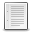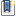File : Details

# rotate3d()

Computes coordinates of rotated points or/and axis, angle, or matrix of a given 3D rotation
Details
Version
1.0
Author
Samuel Gougeon
Maintainer
Samuel Gougeon
Categories
Supported Scilab Version
5.5
Creation Date
August 9, 2015Description
```            rotate3d() computes the new (x', y', z') cartesian coordinates of given points
after rotating them in the 3D space.

The rotation may be specified in 3 ways:
- an example vector and its rotated version are provided.
- a directing vector of the rotation axis, and the rotation angle around it
are provided.
- the rotation matrix is provided.
The rotation center can also be set as an explicit [xc yc zc] vector or to the
Center of Mass of given points. Default is [0 0 0].

According to the way the rotation is specified, rotate3d() can also provide the
unit vector of the rotation axis and the rotation angle, or the rotation
matrix.

Full help is available below as in heading comments in the script.

RESULTS OF EXAMPLES
-------------------

-->// i = unit vector along (Ox), j = along (Oy), k = along (Oz)
-->Rop = rotate3d([2 0 0 ; 0 3 0])  // (Ox)->(Oy)  = +90° around (Oz)
Rop  =
0.    0.    1.    90.

-->Rop = rotate3d([1 1 0 ; 0 0 1])  // (i+j)->(Oz) = +90° around (i-j)
Rop  =
0.7071068  - 0.7071068    0.    90.

-->Rm  = rotate3d([4 0 0 -90]);  // -90° around (+Ox) = {x'=x, y'=z, z'=-y}
-->clean(Rm)
ans  =
1.    0.    0.
0.    0.    1.
0.  - 1.    0.

-->// Rotating the axis of any rotation gives always itself, for any angle:
--> [rx, ry, rz] = rotate3d(2, -1, 5, [2 -1 5 %e]);
--> [rx ry rz]
ans  =
2.    -1.    5.

-->// APPLICATION with a set of points: Animation with a rotated object
-->// ~~~~~~~~~~~~~~~~~~~~~~~~~~~~~~~~
-->// Enter "rotate3d demo" to display the script

------------------------------------------------------------------------------

--> rotate3d

Computes new (x,y,z) cartesian coordinates of points rotated in the 3D space,
or/and the rotation matrix or/and its axis and angle of rotation.

SYNTAXES
--------
rotate3d       // displays this help
rotate3d demo  // display the demo script
Rop              = rotate3d(Rm)
Rm               = rotate3d(Rip)
[Rop, Rm]         = rotate3d(Rip)
[nX, nY, nZ]      = rotate3d(X, Y, Z, Rip [, Rcenter] )
[nX, nY, nZ, Rop, Rm]  = rotate3d(X, Y, Z, Rip [, Rcenter] )

PARAMETERS
----------
X, Y, Z : scalars, vectors, matrices or hypermatrices of cartesian coordinates
of points to be rotated. X, Y, and Z must have the same numbers of
components.

nX,nY,nZ: scalars, vectors, matrices or hypermatrices of cartesian decimal
coordinates of rotated points. nX, nY and nZ take the sizes of X.

Rip  : Rotation Input Parameters = Parameters describing the tri-dimensional
rotation to perform. This argument may have 3 equivalent formats:

a) [a b c phi]: a vector of 4 decimal numbers.
[a b c] are the coordinates of a direction vector of the axis
of the rotation. Not necessarily a unit vector.
phi is the rotation angle around the rotation axis [a b c],
in degrees. Its sign follows the right hand corkscrew rule with
respect to the signed direction [a b c].
Then, we should have, whatever is phi:
[nx,ny,nz]=rotate3d(a,b,c,[a b c phi]); clean([nx,ny,nz]-[a b c])==0

b) [u1 u2 ; v1 v2 ; w1 w2]: a (3,2) matrix of decimal numbers.
The considered rotation turns the [u1 v1 w1] vector into the
[u2 v2 w2] one. The norm of vectors is not taken into account.
The underlying rotation is built such that, if both vectors have
the same norm,
[u2,v2,w2] = rotate3d(u1,v1,w1, [u1 u2; v1 v2;w1 w2])

c) (3,3) rotation matrix.

Rcenter : It may be either a boolean, or a vector of 3 decimal numbers
= coordinates of the rotation center, the point around which
{X,Y,Z} must be rotated:
a) If Rcenter = %t, the Center of Mass of given isoweighted {X,Y,Z}
points is computed and used as rotation center.
b) No indication or Rcenter=%f set Rcenter = [0 0 0]
c) Rcenter = [xc, yc, zc] is used as rotation center.

Rm : (3,3) square real Rotation matrix. Must be orthogonal: (Rm*Rm.'==eye(3,3)
It is such that
[nX(:)-xc  nY(:)-yc  nZ(:)-zc]' =  Rm * [X(:)-xc Y(:)-yc Z(:)-zc]'
whereas the rotation center is located at [xc yc zc].

Rop : Row vector of 7 decimal numbers:
Rop(1:3) = unit vector of the rotation axis. When the rotation is the
identity, its axis is undetermined and [%nan %nan %nan]
is returned.
Rop(4)   = Rotation angle, in degrees.
Rop(5:7) = coordinates of the Center of Mass of the rotated object.
It is set as rotation center when Rcenter=%t is used.

DESCRIPTION
-----------
rotate3d() works in a 3D orthonormal cartesian axes.

Rop = rotate3d(Rm) computes and returns a unit vector Rop(1:3) of the rotation
axis, and the rotation angle Rop(4) around it, corresponding to the
rotation matrix Rm.

Rm = rotate3d(Rip) computes and returns the (3,3) rotation matrix
corresponding to the rotation axis specified by any directing vector
Rip(1:3), for the rotation angle Rip(4).
The rotation matrix always assumes that the rotation center is at [0 0 0].

[Rop, Rm] = rotate3d(Rip) computes and returns the rotation matrix Rm,
the unit vector Rop(1:3) of the rotation axis, and the rotation angle
Rop(4) around it, when the input rotation is specified through an
example of vector and its expected rotated version (w/o respect to norms).

When a set of points to be rotated is specified through their {X, Y, Z}
input coordinates, the coordinates of corresponding rotated points
are as well computed and returned. By default, the origin [0 0 0] is used
as rotation center. Any other center may be explicitly specified through
the Rcenter = [xc yc zc] parameter. When %t is assigned to Rcenter, then
the Center of Mass of the set of points is set as the rotation center.
Its position AFTER the rotation is returned into Rop(5:7).

FEEDBACK
--------

--------
rotate      : Compute rotated coordinates of given points in 2D
rotate_axes : Interactively rotate a graphical Axes
move        : Translate a graphical element in 2D or 3D
scaling     : Apply a 2D homothecy (homogeneous dilatation) to coordinates
of given points.
householder : Symetrize coordinates of given points wrt. a 3D plane (mirror)

EXAMPLES
========
// i = unit vector along (Ox), j = along (Oy), k = along (Oz)
Rop = rotate3d([2 0 0 ; 0 3 0])  // (Ox)->(Oy)  = +90° around (Oz)
Rop = rotate3d([1 1 0 ; 0 0 1])  // (i+j)->(Oz) = +90° around (i-j)
Rm  = rotate3d([4 0 0 -90]);     //-90° around (+Ox) = {x'=x,y'=z,z'=-y}
clean(Rm)

// Rotating the axis of any rotation gives always itself, for any angle:
[rx, ry, rz] = rotate3d(2, -1, 5, [2 -1 5 %e]);
[rx ry rz]  // => [2 -1 5]

// APPLICATION with a set of points: Animation with a rotated object
// ~~~~~~~~~~~~~~~~~~~~~~~~~~~~~~~~
// Enter "rotate3d demo" to display the script

```Files (2)Miscellaneous file
```File defining the function rotate3d()

* MAKE rotate3d() AVAILABLE in EVERY SESSION: Click here to see HOW TO

* Get help: Enter "rotate3d" without parameters
```Screenshot
```Screenshot of the rotate3d() demo for thumbnail
```News (0)Comments (0)Leave a comment
Login withEmail notifications